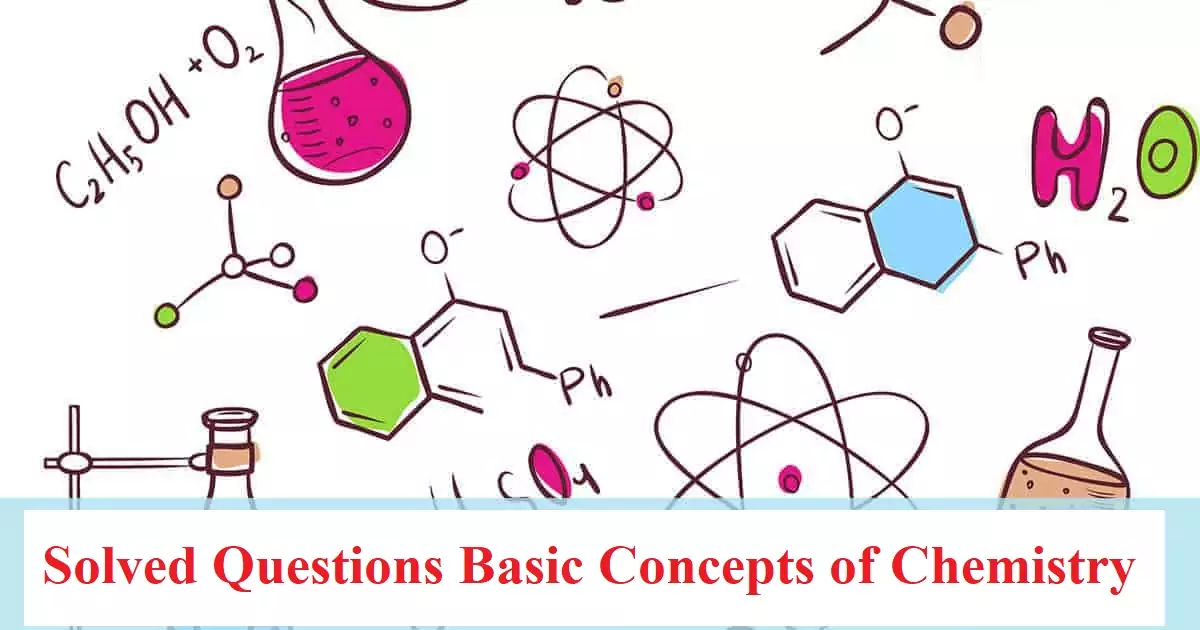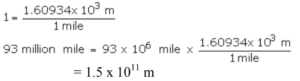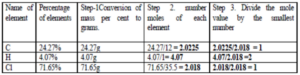# Solved Questions Basic Concepts of Chemistry## Solved Questions Basic Concepts of Chemistry

Solved Questions Basic Concepts of Chemistry

Que 1. How many signiﬁcant ﬁgures are there in (i) 3.070 and (ii) 0.0025?
Ans 1. (i) 4                  (ii) 2

Que 2. How many signiﬁcant ﬁgures are present in the answer of the following calculation:  .0125 + 0.8250 + 0.025
Ans 2.  0.86

Que 3. The density of vanadium is 5.96 g cm3. Express this in the SI unit.
Ans 3. 5960 kg m3

Que 4. The body temperature of a normal healthy person is 370C. Calculate its value in 0F.
Ans 4. We know
0F = 9/5(0C) + 32
0F = 9/5(37) + 32
0F = 9 × 7.4 + 32 = 98.6 0F

Que 5. At what temperature will both the Celsius and Fahrenheit scales read the same value?
Ans 5. Let x be the common reading.
Then, C = F = x
We knoe the relation between 0C and 0F
0C=5/9 (0F-32), and substituting x for 0C and 0F, we get
x =5/9 (x-32)
x =5/9x – 5/9×32
x – 5/9x = 5×32/9
4/9x = – 5×32/9
x = – 5×32/4 = -40
So, -40C = -40F
Hence, at -40 degrees, the readings on the Celsius scale and the Fahrenheit scales are the same.

Que 6. Express the following in the scientiﬁc notation: (i) 0.0048 (ii) 234,000
Ans 6. i). 4.8 × 10-4                ii) 2.34 × 105

Que 7. Given the numbers: 161 cm, 0.161 cm, O.OI61 cm. Write The number of significant figures for these three numbers.
Ans 7. 3,3,3

Que 8. Read the following and choose the incorrect statements.
(i) Both weight and mass are the same quantities used for measurement of the amount of matter present in a substance
(ii) Mass and weight of a substance vary from one place to another due to a change in gravity.
(iii) SI unit of mass is kilogram and while SI unit of weight is the gram.
(a) (i) and (iii)                                     (b) (ii) and (iii)
(c) (i) and (ii)                                      (d) All of these
Ans 8. (d) All of these

Que 9. A student performs a titration with different burettes and finds titre values of 25.2 mL, 25.25 mL, and 25.0 mL the number of significant figures in the average titre value is
a) 1                             b) 3                           c) 2                               d) 4
Ans 9. (b)  Calculate the average value of different titre values.
Then use the rule to determine the significant figures for mathematical calculation.
Average value =  25.2  +  25.25  +  25.0  =   75.45                                                                                                                                   3                             3
= 25.15  = 25.2 mL  The number of significant figure is 3

Que 10. What will be the answer to 77.32-6.3?
a) 71.02                             b) 71.0                        c) 71.2                            d) 71.1
Ans 10. (b)  The answer is to be reported up to the same number of decimal places as that of the term with the least number of decimal places. This after rounding off 71.02 becomes 71.0.

Solved Questions Basic Concepts of Chemistry

Que 11. What is the unit factor method? Express the following in SI units – 93 million miles (distance between earth and sun)
Ans 11. The method to convert units from one system to another is called the unit factor method.
93 million miles = 93 x 106 miles
1 mile = 1.60934 km = 1.60934 x 103 mQue 12. What do mean by gram atomic mass. one million silver atoms weigh 1.79 x 1016 g. Calculate the gram atomic mass of silver.
Ans 12. The atomic mass of an element expressed in grams is the gram atomic mass
Number of silver atoms = 1 million = 1 x 106
Mass of one million Ag atoms = 1.79 x 1016g
Mass of 6.023 x 1023 atoms of Silver = 1.79 x 1016g x 6.023 x 1023                                                                                                                                                                                1 x 106
= 107.8 g

Que 13. Copper oxide obtained by heating copper carbonate or copper nitrate contains copper and oxygen in the same ratio by mass. Which law is illustrated by this observation? State the law.
Ans 13. Law of Definite Proportions: According to this law a chemical compound
always consists of the same elements combined together in the same ratio,
irrespective of the method of preparation or the source from where it is taken.

Que 14. Write the empirical formula of the following:
(a) N2O4                          (b) C6H12O6                               (c) H2O                          (d) H2O2
Ans 14. (a)NO2 (b) CH2O (c) H2O (d) HO

Que 15. Chlorine has two isotopes of atomic mass units 34.97 and 36.97. The relative abundance of the isotopes is 0.755 and 0.245 respectively. Find the average atomic mass of chlorine.
Ans 15. Average atomic mass = 34.97 x 0.755 +36.97 x 0.245 = 35.46 u

Que 16. How are 0.50 mol Na2CO3 and 0.50 M Na2CO3 different?
Ans 16. Molar mass of Na2CO3 = 2 x 23 +12 + 3 x 16 = 106 g / mol
0.50 mol Na2CO3 means 0.50 x 106 = 53 g
0.50 M Na2CO3 means 0.50 mol i.e. 53 g of Na2CO3 are present in I L of the solution.

Que 17. What is the percentage of carbon, hydrogen, and oxygen in ethanol?
Ans 17. The molecular formula of ethanol is: C2H5OH
Molar mass of ethanol is: (212.01 + 61.008 + 16.00) g = 46.068 g
Mass per cent of carbon = (24.02g / 46.068g) ×100 = 52.14%
Mass per cent of hydrogen = (6.048g / 46.068g) × 100 = 13.13%
Mass per cent of oxygen = (16.00 g / 46.068g) ×100 = 34.73%

Que 18. What do mean by molarity? Calculate the molarity of NaOH in the solution
prepared by dissolving its 4 g in enough water to form 250 mL of the solution.
Ans 18. The number of moles of solute dissolved per litre (dm3) of the solution is called molarity.
Since molarity (M) = No. of moles of solute /Volume of the solution in litres
= (Mass of NaOH / Molar Mass of NaOH) /0.250 L
= (4 g / 40 g 0.1 mol) / 0.250L = 0.1 mol / 0.250 L
= 0.4 mol L-1  = 0.4 M

Que 19. Express the following in the scientific notation with 2 significant figures-
(a) 0.0048                                 (b) 234,000                       (c) 200.0
Ans 19. (a) 4.8 x 10-3                       (b) 2.3 x 105                      (c) 2.0 x 102

Que 20. Calculate the number of atoms in each of the following:                                                                  (i) 52 moles of Ar   (ii) 52 u of He         (iii) 52 g of He
Ans 20. (i) 1 mole of Ar = 6.022 × 1023 atoms of Ar
52 mol of Ar = 52 × 6.022 × 1023 atoms of Ar
= 3.131 × 1025 atoms of Ar
(ii) 1 atom of He = 4 u of He
Or
4 u of He = 1 atom of He
1 u of He = 1/4 atom of He
52 u of He = 52/4 atom of He
= 13 atoms of He
(iii) Molar mass of He = 4 g/mol
4 g of He contains = 6.022 × 1023 atoms of He
52 g of He contains = 6.022 × 1023 x 52 = 78.286 x 1023 atoms of He                                                                                                          4

Que 21. What is the difference between empirical and molecular formulas? A compound contains 4.07 % hydrogen, 24.27 % carbon and 71.65 % chlorine. Its molar mass is 98.96 g. What are its empirical and molecular formulas?
Ans 21. An empirical formula represents the simplest whole number ration of various atoms present in a compound whereas the molecular formula shows the exact number of different types of atoms present in a molecule of a compound.The empirical formula of the above compound is CH2Cl.
empirical formula mass is 12 + (1 x 2) + 35.5 = 49.5
n= Molecular Mass / Empirical Formula Mass = 98.96 / 49.5 = 2
Hence molecular formula is C2H4Cl2

Solved Questions Basic Concepts of Chemistry